Electric Potential Energy Formula

Electric Potential Energy Formula

Electric potential energy occurs due to the interaction of two or more charged particles. As the charged particles come close to each other there will be an electrostatic interaction. In the case of two similarly charged particles, the interaction will be to repel (like charges repel). In the case of two oppositely charged particles, the interaction will be to attract (opposites attract).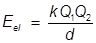Eel = electrostatic potential energy

k =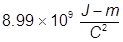Q1 and Q2 = electrical charges on the two particles

d = the distance between the charges

Electric Potential Energy Formula Questions:

1. What is the electrostatic potential energy of two electrons that are 345 nm away from each other?

Note: nm refers to a nanometer or 10-9 m and the charge of an electron is equal to -1.60 x 10-19C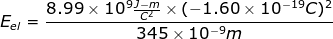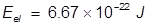2. What is the electrostatic potential of two protons that are 10 cm away from each other?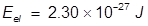Related Links: Electrical Energy to Thermal Energy Conversions Examples Forms of Energy (solar, heat, sound, and motion) Quiz Chemical Energy Facts Electrical Energy Facts Energy Facts Potential Energy Facts Potential Energy Examples Kinetic energy vs. Potential energy Potential Energy: Elastic Formula Potential Energy: Earth's Gravity Formula Potential Energy: Electric Potential Formula Potential Energy: Two-Body Gravitation Formula Potential Energy: Electrostatic Point Particles Formula Elastic Potential Energy Formula